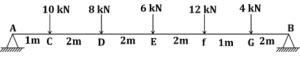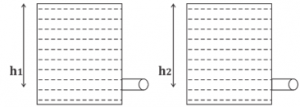Engineering Jobs   »   Civil Engineering Quiz

# GATE’22 CE:- Daily Practice Quiz 26-July-2021

Quiz: Civil Engineering
Exam: GATE
Topic: Miscellaneous

Each question carries 2 marks
Negative marking: 1/3 mark
Time: 12 Minutes

Q1. At the limit state of collapse, an RC beam is subjected to flexural moment 200 kN-m, shear force 20 kN and torque 9 kN-m. the beam is 300 mm wide and has a gross depth of 425 mm, with an effective cover of 25 mm. the equivalent nominal shear stress (τ_ve) as calculated by using the design code turns out to be lesser than the design shear strength (τ_c ) of the concrete. The equivalent flexural moment (M_eq ) for designing the longitudinal tension steel is
(a) 187 kN-m
(b) 200 kN-m
(c) 209 kN-m
(d) 213 kN-m

Q2. A fine-grained soil has liquid limit of 60 and plastic limit of 20. As per the plasticity chart, according to IS Classification, the soil is represented by the letter symbols
(a) CL
(b) CI
(c) CH
(d) CL-ML

Q3. Two small orifices A and B of diameter 1 cm and 2 cm, respectively, are placed on the sides of a tank at depths of h_1 and h_2 below the open liquid surface. If the discharges through A and B are equal, then the ratio of h_1 and h_2 (assuming C_d values)
(a) 16:1
(b) 8: 1
(c) 4:1
(d) 2:1

Q4. The simply supported beam as shown in the figure.Find reaction at support A?
(a) 18 kN
(b) 22 kN
(c) 28 kN
(d) 30 kN

Q5. If a two – dimensional velocity potential function is given by ϕ = 3xy, the discharge between the stream lines passing through (2, 6) and (6, 6) will be:
(a) 14.68 units
(b) 18.97 units
(c) 25.45 units
(d) 48 units

Solutions

S1. Ans.(d)
Sol. Given,
M = 200 kN – m
T = 9 kN-m
D = 425 mm
B = 300 mm
Meq = ?
Meq = M +T/1.7 (1+D/B)

=200+9/1.7 (1+425/300)
=212.79≈213 kN-m

S2. Ans.(c)
Sol. w_L=60
w_P=20
Plasticity index (I_P)=w_L -w_P
= 60 – 20
= 40
Equation of A – line →
(I_P )_(A-line )=0.73 (W_L-20 )
= 0.73 (60 – 20)
= 29.2
(I_P )>(I_P )_(A-line), thus, the given soil lies above A – line & w_L is more than 50, hence soil will be CH.

S3. Ans.(a)
Sol.Q_A=Q_B
A_1 V_1=A_2 V_2
π/4 D_1^2 (√(2gh_1 ))=π/4 D_2^2 (√(2gh_2 ))
h_1/h_2 =[D_2/D_1 ]^4
h_1/h_2 =[2/1]^4
h_1:h_2=16:1

S4. Ans.(b)
Sol. R_A+R_B=40 kN
Taking moment about point A →
ΣM_A=0
R_B×10-4×8-12×7-6×5-8×3-10×1=0
10R_B-32-84-30-24-10=0
10 R_B=180
▭(R_B=18 kN)
Reaction at A = (R_A ) = 40 – R_B
= 40 – 18
▭(R_A=22 kN)

S5. Ans.(d)
Sol. Given,
ϕ = 3xy
(-∂ϕ)/∂x=u= -3y
(-∂ϕ)/∂y=v =-3x
Now, for stream function
u=(-∂Ψ)/∂y
-3y=(-∂Ψ)/∂y
∂Ψ=3y.∂y
⎰∂Ψ= ⎰3y.∂y
▭(Ψ_1=3/2 y^2 )
v=∂Ψ/∂x
-3x=∂Ψ/∂x
∂Ψ= -3x.∂x
▭(Ψ_2= -3/2 x^2 )
Ψ= Ψ_1+Ψ_2
=3/2 y^2-3/2 x^2
Ψ=3/2 (y^2-x^2 )
Now,
Ψ_1 (2,6)=3/2 [(6)^2-(2)^2 ]=48 units
Ψ_2 (6,6)=3/2 [(6)^2-(6)^2 ]=0 units
Discharge (Q) = |Ψ_1- Ψ_2 |
=|48-0|
= 48 units

Sharing is caring!